# Exploratory Regression Shiny App

#### 2018-07-24

The Exploratory Regression Shiny App (ERSA) package consists of a collection of functions for displaying the results of a regression calculation, which are then packaged together as a shiny app function.

To use ERSA first do

``library(ERSA)``

Then construct a regression model of your choice.

``````f <- lm(Fertility ~ . , data = swiss)
exploreReg(f,swiss)``````

Here is a screen shot of the result: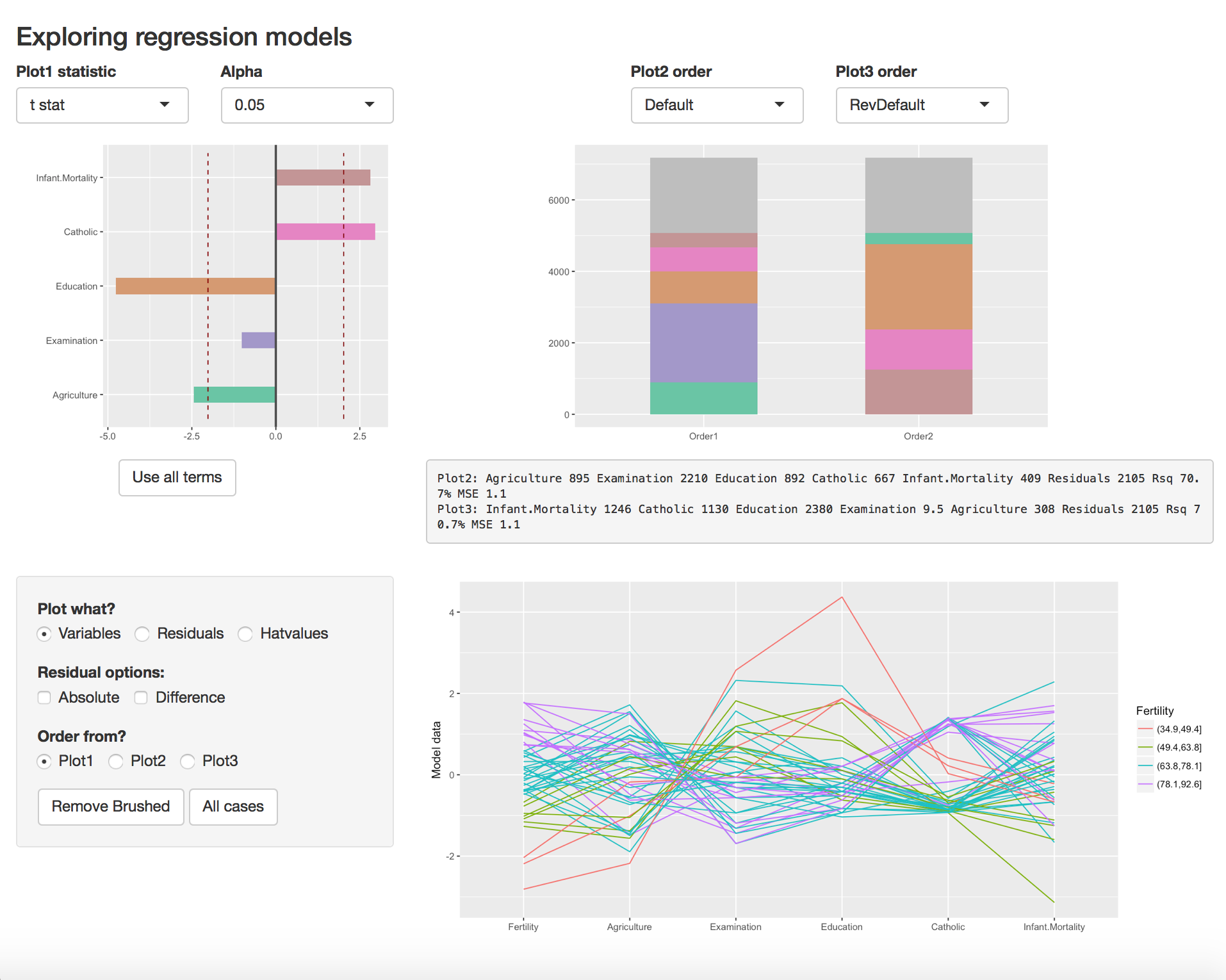## The summary or drop1 display

The app display consists of four panels. In the top left corner is a display of the model summary t-statistics, from

``````f <- lm(Fertility ~ . , data = swiss)
summary(f)``````
``````##
## Call:
## lm(formula = Fertility ~ ., data = swiss)
##
## Residuals:
##      Min       1Q   Median       3Q      Max
## -15.2743  -5.2617   0.5032   4.1198  15.3213
##
## Coefficients:
##                  Estimate Std. Error t value Pr(>|t|)
## (Intercept)      66.91518   10.70604   6.250 1.91e-07 ***
## Agriculture      -0.17211    0.07030  -2.448  0.01873 *
## Examination      -0.25801    0.25388  -1.016  0.31546
## Education        -0.87094    0.18303  -4.758 2.43e-05 ***
## Catholic          0.10412    0.03526   2.953  0.00519 **
## Infant.Mortality  1.07705    0.38172   2.822  0.00734 **
## ---
## Signif. codes:  0 '***' 0.001 '**' 0.01 '*' 0.05 '.' 0.1 ' ' 1
##
## Residual standard error: 7.165 on 41 degrees of freedom
## Multiple R-squared:  0.7067, Adjusted R-squared:  0.671
## F-statistic: 19.76 on 5 and 41 DF,  p-value: 5.594e-10``````

This display (Plot1) shows the magnitude of each t-statistic. The red dashed line shows the 5% significance level. There are a few other options for this display. The display may be switched to “CI”, which shows parameter confidence intervals or “CI stdX” for confidence intervals in standard X units. If the model contains factors with more than two levels, then better choices are “F stat” or “Adj. SS”. These give the summaries from the Sum of Sq or F stat columns of the drop1 results:

``drop1(f, test="F")``
``````## Single term deletions
##
## Model:
## Fertility ~ Agriculture + Examination + Education + Catholic +
##     Infant.Mortality
##                  Df Sum of Sq    RSS    AIC F value    Pr(>F)
## <none>                        2105.0 190.69
## Agriculture       1    307.72 2412.8 195.10  5.9934  0.018727 *
## Examination       1     53.03 2158.1 189.86  1.0328  0.315462
## Education         1   1162.56 3267.6 209.36 22.6432 2.431e-05 ***
## Catholic          1    447.71 2552.8 197.75  8.7200  0.005190 **
## Infant.Mortality  1    408.75 2513.8 197.03  7.9612  0.007336 **
## ---
## Signif. codes:  0 '***' 0.001 '**' 0.01 '*' 0.05 '.' 0.1 ' ' 1``````

Clicking on this display removes the closest predictor, clicking again adds it in. Once a predictor is added or dropped, this change is also reflected in the other ERSA displays.

## The anova display

The second panel in the top right shows the results of

``anova(f)``
``````## Analysis of Variance Table
##
## Response: Fertility
##                  Df  Sum Sq Mean Sq F value    Pr(>F)
## Agriculture       1  894.84  894.84 17.4288 0.0001515 ***
## Examination       1 2210.38 2210.38 43.0516 6.885e-08 ***
## Education         1  891.81  891.81 17.3699 0.0001549 ***
## Catholic          1  667.13  667.13 12.9937 0.0008387 ***
## Infant.Mortality  1  408.75  408.75  7.9612 0.0073357 **
## Residuals        41 2105.04   51.34
## ---
## Signif. codes:  0 '***' 0.001 '**' 0.01 '*' 0.05 '.' 0.1 ' ' 1``````

as Plot2. Each predictor of this output is represented by a coloured slice, whose height represents the sum of squares. These sums of squares depend on the order in which predictors were entered into the model fit. The second barchart (Plot3) represents the anova table obtained by reversing the predictor order. The dropdown menus give other choices. Forwards and backwards give the order of predictors as visited by the forwards and backwards selection algorithms. By choosing order “Random”, a user can click on a slice to move it up one position, or double click to move it down.

## The Parallel coordinates plot display

The lower part of the app has a parallel coordinate plot on the lower right, and a control panel on the left. The user can choose to show either (i) Variables (ii) Residuals and (iii) Hatvalues. Each axis is assigned to a variable, using the order from Plot1, Plot2 and Plot3. The residuals and hatvalues are obtained by leaving out one predictor at a time, if the selected order is Plot1, or by adding predictors in sequence if the order is Plot2 or Plot3. When residuals are plotted, the Difference option when selected shows the difference

Specifically, let \(e\) denote the vector of residuals from the full model and let \(e^{-j}\) denote the residual vector from the fit using all predictors except the \(j\)th. When residuals are selected, using order from Plot1, the PCP shows \(e^{-j}\) on the \(j\)th axis, or \(e^{-j} - e\) when the Difference button is selected in Residual options. Let \(e^{j}\) be the residuals from the model including the first \(j\) predictors. When Residuals are selected, using order from Plot2 or Plot3, the PCP shows \(e^{j}\) on the \(j\)th axis, or \(e^{j} - e^{j-1}\) when the Difference button is selected in Residual options. For any of the Plot orders, when the Absolute button is selected, either absolute residuals or absolute residual differences are plotted.

Dragging a brush over the PCP axes will highlight cases and print information for the selected cases. Clicking on Remove Brushed will remove the highlighted cases from view. All regression models are recalculated and the displays are updated. Clicking the All Cases buttom will update all displays to use the complete dataset. Double clicking on the PCP itself will un-highlight all cases.

## Individual plots

There are functions to construct static plot versions of all the plots in the Exploratory Regression shiny app.

For the Plot1 displays use

``plottStats(f)``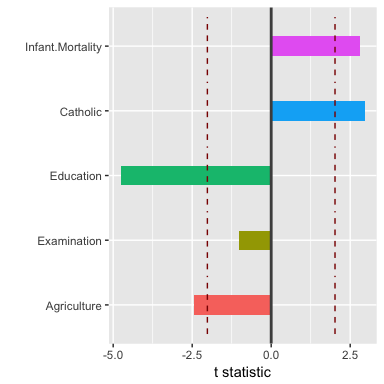``````cols <- termColours(f)
plottStats(f, cols)``````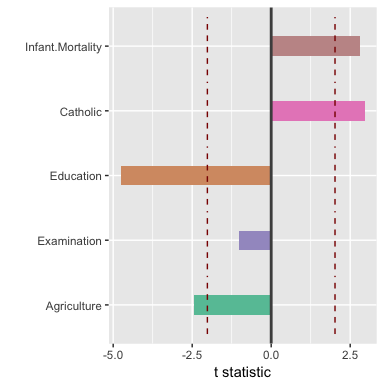or

``````plotCIStats(f,cols)
plotCIStats(f, cols,stdunits=TRUE)
plotAnovaStats(f, cols,type="F")
plotAnovaStats(f, cols,type="SS")``````

For the Plot2 displays use

``````fr <- revPredOrd(f, swiss)
plotSeqSS(list(f,fr), cols,legend=TRUE)``````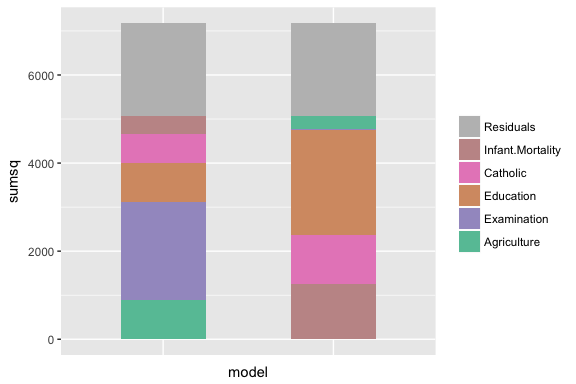Other orders are

``````fselOrder(f)
bselOrder(f)
randomPredOrder(f)
regsubsetsOrder(f)``````

To plot the PCP display of the data use:

``pcpPlot(swiss, f)``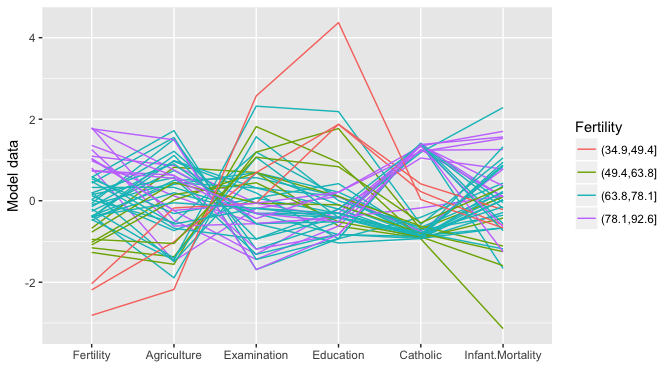Cases are automatically coloured by the magnitude of the response.

To plot a PCP display of the residuals leaving out one predictor at a time use

``pcpPlot(swiss, f, type="Residuals")``